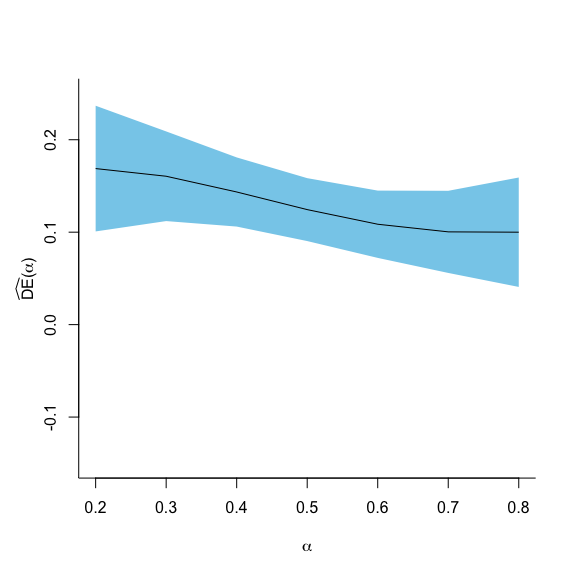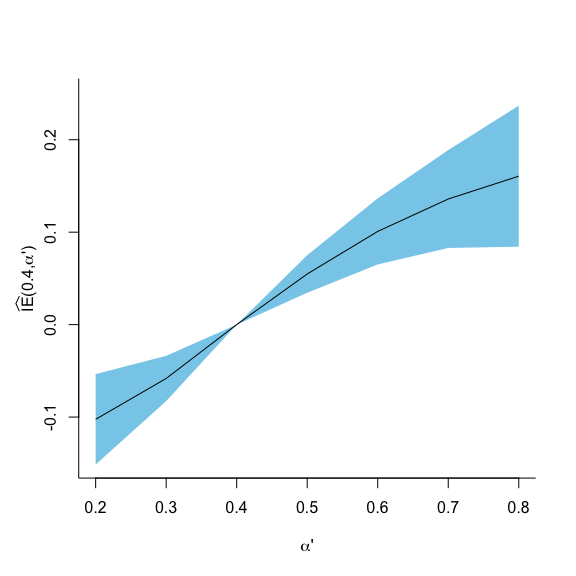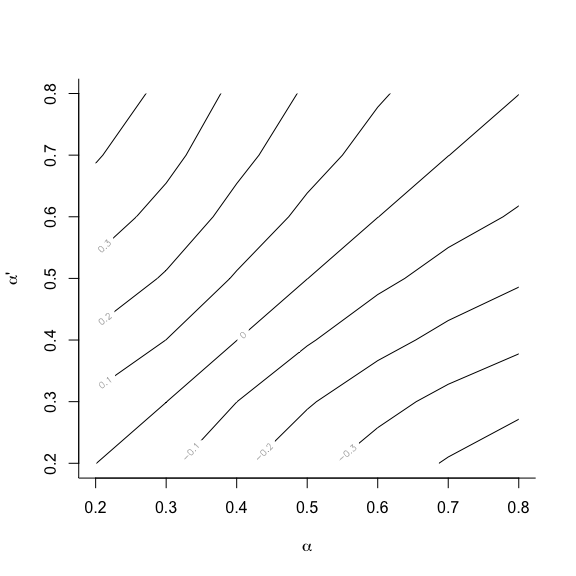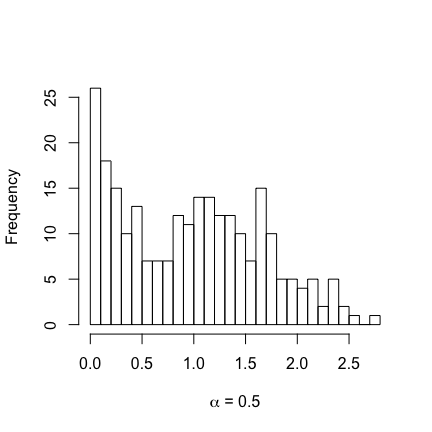# inferference Vignette

University of North Carolina
University of North Carolina

# JSS article

For a complete overview of the software, please refer to the Journal of Statistical Software paper: “A Recipe for inferference: Start with Causal Inference. Add Interference. Mix Well with R.”

# Using inferference

## User’s guide

The list below details the arguments for interference, the primary function in inferference. Special attention should be given to the propensity_integrand and formula arguments.

• formula: formula used to define the causal model. formula has a minimum of 4 parts, separated by | and ~ in a specific structure: outcome | exposure ~ covariates | group. The order matters, and the pipes (|) split the data frame into corresponding pieces . The exposure ~ covariates piece is passed as a single formula to the chosen model_method (defined below) used to estimate or fix propensity parameters.
• The following includes a random effect for the group: outcome | exposure ~ covariates + (1|group) | group. In this instance, the group variable appears twice.
• If the study design includes a “participation” variable (as in both examples below), this is easily added to the formula: outcome | exposure | participation ~ covariates | group.
• propensity_integrand: a function, which may be created by the user, used to compute the IP weights. This defaults to the function logit_integrand(), which calculates the product of inverse logits for individuals in a group: $$\prod_{j = 1}^{n_i} \{r h_{ij}(b_i)\}^{A_{ij}}\{1 - r h_{ij}(b_i)\}^{1 - A_{ij}} f_b(b_i; \theta_s)$$, where $$h_{ij}(b_i) = \mbox{logit}^{-1}(\mathbf{X}_{ij}\theta_x + b_i)$$, $$b_i$$ is a group-level random effect, $$f_b$$ is a $$N(0, \theta_s)$$ density, and $$r$$ is a known constant. In an observational study typically $$r = 1$$. The examples below include individual randomized experiments in which case $$r$$ denotes the randomization probability among trial participants. logit_integrand() is the integrand of , logit_integrand() ignores the random effect. IP weights are computed by numerically integrating propensity_integrand over the random effect distribution using by stats::integrate() to which arguments may be passed via ... (see below). The default logit_integrand() also takes the following argument that can be passed via the ... argument in interference():
• randomization: a scalar. This is the $$r$$ in the formula just above. It defaults to 1 in the case that a participation vector is not included. The vaccine study example below demonstrates use of this argument.
• loglihood_integrand: a function, which may be created by the user, that defines the log likelihood of the propensity score model. This should generally be the same function as propensity_integrand}, which is the default.
• allocations: a vector of values in $$[0, 1]$$. Increasing the number of elements of the allocation vector increases computation time; however, a larger number of allocations will make plotted effect estimates smoother. A minimum of two allocations is required.
• data: the analysis data frame. This must include all the variables defined in the formula}.
• model_method: the method used to estimate or set the propensity model parameters. Must be one of 'glm', 'glmer', or 'oracle'. For a fixed effects only model use 'glm', or to include random effects use lme4’s 'glmer' (Bates et al. 2014). logit_integrand only supports a single random effect for the grouping variable, corresponding to $$b_i$$. When the propensity parameters are known (as in simulations) or if estimating parameters for the propensity model outside of interference, use the 'oracle' option. See model_options for details on how to pass the oracle parameters. Defaults to 'glmer'}.
• model_options: a list of options passed to the function in model_method. Defaults to list(family = binomial(link = 'logit')). When model_method = 'oracle', the list must have two elements, fixed.effects and random.effects. If the model does not include random effects, set random.effects = NULL.
• causal_estimation_method: currently only supports and defaults to 'ipw'.
• causal_estmation_options: a list with a single item variance_estimation, which is either 'naive' or 'robust'. Defaults to 'robust'.
• conf.level: level for confidence intervals. Defaults to 0.95.
• rescale.factor: a scalar multiplication factor by which to rescale outcomes and effects. Defaults to 1.
• integrate_allocation: indicator of whether the integrand function uses the allocation parameter. Defaults to TRUE.
• ...: used to pass additional arguments to internal functions such as numDeriv::grad() or stats::integrate(). Arguments can also be passed to the propensity_integrand and loglihood_integrand functions.

## The interference object

An interference() call results in an S3 object of class interference which contains:

• estimates: a data frame of causal effect estimates;
• models$propensity_model: the glm or glmer object; • summary: a list of objects summarizing the causal model such as the number of groups, number of allocations, and the formula used in the interference call; • weights: (# of groups) $$\times$$ (# of allocations) matrix of group-level weights: $w_{i, k} = \frac{\pi_i(\mathbf{A}_i; \alpha_k)}{f_{\mathbf{A}_i|\mathbf{X}_i}(\mathbf{A}_i|\mathbf{X}_i; \hat{\boldsymbol{\theta}})}.$ If variance_estimation = 'robust', then the object also includes: • weightd: (# of groups) $$\times$$ (# of allocations) $$\times$$ (# of parameters) array of weights computed using derivatives of the propensity function with respect to each parameter; • scores: (# of groups) $$\times$$ (# of parameters) matrix of derivatives of the log likelihood. ## Utility functions The package includes tools to extract effect estimates of interest from the S3 object. The functions direct_effect, indirect_effect (or ie), total_effect (or te), and overall_effect (or oe) select appropriate records from the estimates data frame in the interference object. # Example: Vaccine Study This section illustrates the use of inferference with an example drawn from vaccine research. The package includes a single dataset based on the same set of parameters used in the simulation study by Perez-Heydrich et al. (2014). The vaccinesim dataset consists of 3000 units in 250 groups and contains two covariates (X1 = age in decades and X2 = distance to river), a vaccination indicator (A), a participation indicator (B), a binary outcome (Y) indicating cholera infection (1 yes, 0 no), and the unit’s group. > library(inferference) > head(vaccinesim) ## Y X1 X2 A B group ## 1 1 5.3607405 1.715527 0 0 1 ## 2 0 0.1964597 1.730802 0 1 1 ## 3 0 0.4846243 1.769546 1 1 1 ## 4 0 0.8012977 1.715527 0 1 1 ## 5 0 2.1426629 1.772158 1 1 1 ## 6 0 1.2861017 1.715527 0 1 1 Like the original study (M. Ali et al. 2005) that inspired the simulation, individuals were randomized to vaccine with a known probability of $$2/3$$, but subjects could opt to not participate in the trial. In essence, there are both experimental and observational aspects to the data. The interference function handles this design when logit_integrand’s randomization argument is used and a participation variable is included in the formula. > example1 <- interference( + formula = Y | A | B ~ X1 + X2 + (1|group) | group, + allocations = c(.3, .45, .6), + data = vaccinesim, + randomization = 2/3, + method = 'simple') The only arguments required for interference to run are formula, allocations, and data. When using the robust' method (the default) to compute the variance, the internal workings call numDeriv::grad (Gilbert and Varadhan 2012) and stats::integrate frequently. The option method = 'simple' greatly speeds up the numDeriv::grad function. For more accurate derivatives, leave out this option. See ?numDeriv::grad for more options. The print.interference function provides an overview of the causal effect estimates, estimated standard errors, and Wald-type confidence intervals. In the output, alpha1 and alpha2 refer to $$\alpha$$ and $$\alpha'$$, while trt1 and trt2 refer to $$a_1$$ and $$a_2$$, respectively. > print(example1) ## -------------------------------------------------------------------------- ## Model Summary ## -------------------------------------------------------------------------- ## Formula: Y | A | B ~ X1 + X2 + (1 | group) | group ## Number of groups: 250 ## 3 allocations were used from 0.3 (min) to 0.6 (max) ## -------------------------------------------------------------------------- ## Causal Effect Summary ## Confidence level: 0.95 ## Variance method: robust ## -------------------------------------------------------------------------- ## ## Direct Effects ## alpha1 trt1 alpha2 trt2 estimate std.error conf.low conf.high ## 0.30 0 0.30 1 0.1605 0.02474 0.11202 0.2090 ## 0.60 0 0.60 1 0.1086 0.01857 0.07219 0.1450 ## 0.45 0 0.45 1 0.1339 0.01778 0.09904 0.1687 ## ## Indirect Effects ## alpha1 trt1 alpha2 trt2 estimate std.error conf.low conf.high ## 0.30 0 0.60 0 0.15907 0.02617 0.10777 0.2104 ## 0.30 0 0.45 0 0.08657 0.01732 0.05263 0.1205 ## 0.45 0 0.60 0 0.07250 0.01408 0.04490 0.1001 ## ## Total Effects ## alpha1 trt1 alpha2 trt2 estimate std.error conf.low conf.high ## 0.30 0 0.60 1 0.2677 0.02435 0.2199 0.3154 ## 0.30 0 0.45 1 0.2205 0.02469 0.1721 0.2688 ## 0.45 0 0.60 1 0.1811 0.01841 0.1450 0.2172 ## ## Overall Effects ## alpha1 trt1 alpha2 trt2 estimate std.error conf.low conf.high ## 0.30 NA 0.60 NA 0.17607 0.019247 0.13835 0.2138 ## 0.30 NA 0.45 NA 0.09867 0.014207 0.07083 0.1265 ## 0.45 NA 0.60 NA 0.07740 0.008981 0.05980 0.0950 ## ## -------------------------------------------------------------------------- The utility functions return selected effect estimates. > direct_effect(example1, .3) ## alpha1 trt1 alpha2 trt2 estimate std.error conf.low conf.high ## 1 0.3 0 0.3 1 0.1605036 0.02473782 0.1120184 0.2089888 > ie(example1, .3) ## alpha1 trt1 alpha2 trt2 estimate std.error conf.low conf.high ## 1 0.3 0 0.30 0 0.00000000 0.00000000 0.00000000 0.0000000 ## 2 0.3 0 0.45 0 0.08656992 0.01731878 0.05262574 0.1205141 ## 3 0.3 0 0.60 0 0.15906887 0.02617398 0.10776882 0.2103689 ## Plotting effect estimates Plots of effect estimates over a range of $$\alpha$$ levels may be helpful in summarizing results. Perez-Heydrich et al. (2014) present several such graphical displays. Here we demonstrate how to generate similar plots of effect estimates using inferference. First, we estimate the effects over a dense sequence of allocations so that lines will be smooth. > example2 <- interference( formula = Y | A | B ~ X1 + X2 + (1|group) | group, + allocations = seq(.2, .8, by = .1), + data = vaccinesim, randomization = 2/3, method = 'simple') In the plots, we present the direct and indirect effect estimates over this range of allocations. For direct effects, a simple scatterplot showing the point-wise confidence intervals suffices. One approach with indirect effects fixes $$\alpha$$ and plots estimates over a range of $$\alpha'$$, whereas a contour plot displays all pairwise $$(\alpha, \alpha')$$ comparisons over a range of allocation strategies. > deff <- direct_effect(example2) > x <- deff$alpha1
> y <- as.numeric(deff$estimate) > u <- as.numeric(deff$conf.high)
> l <- as.numeric(deff$conf.low) > plot(c(min(x), max(x)), c(-.15, .25), type = 'n', bty = 'l', + xlab = expression(alpha), ylab = '' ) > title(ylab = expression(widehat(DE) * "(" * alpha * ")"), + line = 2) > polygon(c(x, rev(x)), c(u, rev(l)), col = 'skyblue', border = NA) > lines(x, y, cex = 2)> ieff.4 <- ie(example2, allocation1 = .4) > x <- ieff.4$alpha2
> y <- as.numeric(ieff.4$estimate) > u <- as.numeric(ieff.4$conf.high)
> l <- as.numeric(ieff.4$conf.low) > plot(c(min(x), max(x)),c(-.15, .25), type = 'n', bty = 'l', + xlab = expression(alpha * "'"), ylab = '') > title(ylab = expression(widehat(IE) * "(" * 0.4 * "," * alpha * "'" * ")"), + line = 2) > polygon(c(x, rev(x)), c(u, rev(l)), col = 'skyblue', border = NA) > lines(x, y, cex = 2)> ieff <- subset(example2[["estimates"]], effect == 'indirect') > x <- sort(unique(ieff$alpha1))
> y <- sort(unique(ieff$alpha2)) > z <- xtabs(estimate ~ alpha1 + alpha2, data= ieff) > contour(x, y, z, xlab = expression(alpha), + ylab = expression(alpha * "'"), bty = 'l')## Diagnostics IPW estimators are known to be unstable if the weights range greatly. The package includes a basic utility to check the performance of the group-level weights, $$w_{i,k}$$, for multiple allocations. The function diagnose_weights plots histograms of weights for chosen allocation levels. If the allocations argument is left NULL, the function plots histograms for five allocation levels used the interference call. The plot below shows the resulting histogram for a single allocation. The analyst should examine groups with extreme weights, which may unduly influence population-level estimates. > diagnose_weights(example2, allocations = .5, breaks = 30)# Example: Voting Experiment The preceding example used the default logit_integrand function to define the group-level propensities. The following example demonstrates how to customize the propensity score function. Nickerson (2008) reported an experiment on voter behavior to examine peer-to-peer indirect effects on voting participation. The experiment randomized households with only two registered voters in Denver and Minneapolis to receive one of three assignments: voting encouragement, recycling encouragement, or nothing. Canvassers knocked on doors of households randomized to the voting or recycling groups a week before the 2002 primary. If a registered voter answered the door, the canvassers delivered a scripted message about voting (treatment) or recycling (control). The researchers used voter turnout records to determine if each member of the household then voted in the election. Nickerson was interested in the potential spillover effect of the voting encouragement to the untreated individual via the treated individual. From analysis of the observed data, he concluded there was a “secondary effect” where the household members not contacted by the canvassers voted more often in the treatment groups compared to the control groups. The dataset voters contains information for 3861 households, 2549 in Denver and 1312 in Minneapolis, including covariates such as age, gender, previous voting history, and party affiliation. Our estimand of interest involves average voting outcomes when households receive voting encouragement compared to when household receive the recycling message, hence we exclude households not contacted by canvassers. We also exclude the single household where a canvasser appears to have contacted both registered voters. > voters <- within(voters, { + treated = (treatment == 1 & reached == 1) * 1 + c_age = (age - mean(age))/10 + }) > reach_cnt <- tapply(voters$reached, voters$family, sum) > voters <- voters[!(voters$family %in% names(reach_cnt[reach_cnt > 1])), ]
> voters <- voters[voters\$hsecontact == 1, ]

## Household-level propensity

Unlike the vaccine study example, in this data set randomization occurred at the group level but individual level treatment was not randomized. With only two subjects, $$\mathbf{A}_i = (A_{i1}, A_{i2})$$ is the treatment allocation for group $$i$$ and $$\mathbf{X}_i = (\mathbf{X}_{i1}, \mathbf{X}_{i2})$$ is the matrix of individuals’ covariate matrices for group $$i$$. Let $$\mathbf{B}_i = (B_{i1}, B_{i2})$$ be indicators of being reached by a canvasser in group $$i$$. Since we only consider households where someone answered the door, $$\mathbf{B}_i \in \{(1, 0), (0, 1)\}$$ and $$\Pr(B_{i1} = 1| \mathbf{X}_{i}) + \Pr(B_{i2} = 1| \mathbf{X}_{i}) = 1$$. Let $$h_{ij} = \Pr(B_{ij} = 1| \mathbf{X}_{i}; \theta) = \mbox{logit}^{-1}(\mathbf{X}_{i}\theta_x)$$. Let $$G_i \in \{0, 1\}$$ be the indicator that group $$i$$ is randomized to treatment (1) or control (0). By design, $$\Pr(G_i = 1) = 0.5$$. Since $$\Pr(A_{i1} | \mathbf{X}_i; \theta) = 1 - \Pr(A_{i2} | \mathbf{X}_i; \theta)$$, $$\Pr(\mathbf{A}_i | \mathbf{X}_i; \theta)$$ can arbitrarily be defined in terms of either household member. By convention we use the first subject (subject one) of each group found in the dataset. Among treated groups, the probability of subject one being treated is the probability that a canvasser reached subject one. That is, $$\Pr(A_{i1} | G_{i} = 1, \mathbf{X}_i; \theta) = h_{i1}^{A_{i1}}(1 - h_{i1})^{1 - A_{i1}}$$. Thus, the group-level propensity can be expressed:

\begin{align*} \Pr(\mathbf{A}_i | \mathbf{X}_i ; \theta) &= \Pr(\mathbf{A}_i | G_i = 1, \mathbf{X}_i ; \theta)\Pr(G_i = 1) + \Pr(\mathbf{A}_i | G_i = 0, \mathbf{X}_i ; \theta)\Pr(G_i = 0) \\ &= 0.5 \{ \Pr(\mathbf{A}_i | G_i = 1, \mathbf{X}_i ; \theta) + \Pr(\mathbf{A}_i | G_i = 0, \mathbf{X}_i ; \theta) \} \\ &= \begin{cases} .5 & \text{if A_{i1} = 0, A_{i2} = 0 and G_i = 0} \\ .5 h_{i1}^{A_{i1}}(1 - h_{i1})^{1 - A_{i1}} & \text{if (A_{i1} = 0, A_{i2} = 1 or A_{i1} = 1, A_{i2} = 0) and G_i = 1} \\ 0 & \text{otherwise} \end{cases} \\ \end{align*}

Thus, $$h_{i1}$$ is sufficient to determine the group-level propensity. If we know whether or not the first subject was reached by a canvasser, then we know if the second was. Therefore, we can estimate parameters for $$h_{i1}$$ with a dataset that includes only subject one from each group. To do this, we must estimate the parameters outside of inferference and use model_method = 'oracle'. We include centered age (in decades) in the propensity model for demonstration purposes.

> voters1 <- do.call(rbind, by(voters, voters[, 'family'], function(x) x[1, ]))
> coef.voters <- coef(glm(reached ~ c_age, data = voters1,
+                      family = binomial(link = 'logit')))

## Coding the propensity function

Custom propensity_integrand and loglihood_integrand functions must have at least one argument:

• b: the first argument is the variable for which the integrate function computes the integral. As in this example, the function can be written so that the integral evaluates to 1 and has no effect.

For example, the following function will fix the group-level propensity to 0.5 for all groups when variance_estimation = "naive":

> fixed_propensity <- function(b){
+   return(0.5 * dnorm(b))
+ }

For more realistic models, additional arguments may be passed to the custom function:

• X: the covariate matrix (determined by the formula}) for the $$i$$th group
• A: the vector of treatment indicators for the $$i$$th group
• parameters: vector of estimated parameters from the model_method * allocation: the allocation level for which the propensity is currently being estimated
• ...: other arguments can be passed via the ellipsis in interference Now we have the pieces to write the propensity function for the voting example.
> household_propensity <- function(b, X, A,
+                                  parameters,
+                                  group.randomization = .5){
+   if(!is.matrix(X)){
+     X <- as.matrix(X)
+   }
+   if(sum(A) == 0){
+     pr <-  group.randomization
+   } else {
+     X.1 <- X[1 ,]; A.1 <- A
+     h   <- plogis(X.1 %*% parameters)
+     pr  <-  group.randomization * dbinom(A.1, 1, h)
+   }
+   out <- pr * dnorm(b)
+   out
+ }

## Evidence of a peer influence effect

The influence of the door opener on the non-door opener’s voting behavior corresponds to an indirect effect. Though the Bernoulli-type parametrization of the estimands allows us to look at a range of allocations, $$\widehat{IE}(0.5, 0)$$ makes the sensible comparison between a world where individuals receive a voting message with probability $$0.5$$ to a world where individuals have zero probability of receiving the voting message.

> example3 <- interference(
+   formula = voted02p | treated | reached ~ c_age | family,
+   propensity_integrand = 'household_propensity',
+   data = voters,
+   model_method = 'oracle',
+   model_options = list(fixed.effects = coef.voters, random.effects = NULL),
+   allocations   = c(0, .5),
+   integrate_allocation = FALSE,
+   causal_estimation_options = list(variance_estimation = 'robust'),
+   conf.level = .9)
> ie(example3, .5, 0)[ , c('estimate', 'conf.low', 'conf.high')]
##     estimate    conf.low  conf.high
## 1 0.03151501 0.002290586 0.06073944

The point estimate suggests an individual receiving the voting encouragement increases the voting likelihood of the other household member by 3.2%. The 90% confidence interval excludes zero, indicating a significant indirect effect corroborating the analysis in Nickerson (2008).

For comparison, suppose that a flip of a fair coin determined which registered voter opened the door. We exclude age as a covariate and instead set $$h_{i1} = 0.5$$. Here we assume to know the propensity score, so we use variance_estimation = 'naive'.

> example4 <- interference(
+   formula = voted02p | treated | reached ~ 1 | family,
+   propensity_integrand = 'household_propensity',
+   data = voters,
+   model_method = 'oracle',
+   model_options = list(fixed.effects = 0, random.effects = NULL),
+   allocations   = c(0, .5),
+   integrate_allocation = FALSE,
+   causal_estimation_options = list(variance_estimation = 'naive'),
+   conf.level = .9)
> ie(example4, .5, 0)[ , c('estimate', 'conf.low', 'conf.high')]
##     estimate    conf.low  conf.high
## 1 0.03144654 0.002209019 0.06068406

Examining the group-level weights may help diagnose coding errors in the propensity score function. In the case of a fixed probability as in example4}, the propensity weights are easily computed by hand. For example, for $$\alpha = 0.5$$,

$w_i = \frac{\pi(\mathbf{A}_i; 0.5)}{f_{\mathbf{A}_i | \mathbf{X}_i}(\mathbf{A}_i | \mathbf{X}_i; \theta = 0)} = \begin{cases} \frac{0.5^2}{.5} = .5 & \text{ if } G_i = 0 \\ \frac{0.5^2}{.5^2} = 1 & \text{ if } G_i = 1\\ \end{cases},$

which we can confirm the software computed.

> G <- tapply(voters[1:12, 'treated'], voters[1:12, 'family'], sum)
> W <- head(example4[["weights"]])[, 2]
> cbind(G, W)
##    G   W
## 2  1 1.0
## 4  0 0.5
## 5  0 0.5
## 6  0 0.5
## 9  1 1.0
## 10 0 0.5

# Computational Issues with IPW Estimators

We show in this section how computation of the group-level weights may affect estimation as the number of individuals in groups grows. To illustrate, consider the IPW estimator of the overall effect, which weights individual outcomes in group $$i$$ with:

$w_{1i} = \frac{\pi(\textbf{A}_i ; \alpha)}{f_{\mathbf{A}_i | \mathbf{X}_i}(\mathbf{A}_i | \mathbf{X}_i; \hat{\boldsymbol{\theta}})} = \frac{\prod_{j=1}^{n_i} \alpha^{A_{ij}} (1 - \alpha)^{1 - A_{ij}}}{ \int \prod_{j=1}^{n_i} h_{ij}^{A_{ij}} (1 - h_{ij})^{1 - A_{ij}} f_b (b_i ; \hat{\theta}_s) db_i }$

or equivalently, $w_{2i} = \left\{ \int \prod_{j=1}^{n_i} \left(\frac{h_{ij}}{\alpha}\right)^{A_{ij}} \left(\frac{1 - h_{ij}}{1 - \alpha}\right)^{1 - A_{ij}} f_b (b_i ; \hat{\theta}_s) db_i \right\}^{-1}$

or, $w_{3i} = \left\{ \int \exp\left[ \sum_{j=1}^{n_i} \left\{ A_{ij} \log \left( \frac{h_{ij}}{\alpha}\right) + (1 - A_{ij})\log \left(\frac{1 - h_{ij}}{1 - \alpha}\right) \right\} \right] f_b (b_i ; \hat{\theta}_s) db_i \right\}^{-1}.$

While mathematically equivalent, these weights may be computationally dissimilar. In the case of $$w_{1i}$$, the product term within the integral entails multiplying probabilities and thus will tend to 0 as $$n_i$$ increases, causing the denominator of $$w_{1i}$$ to get arbitrarily large. In contrast, the product term in $$w_{2i}$$ entails multiplying values which may be less than or greater than 1 and thus tends to be less susceptible to numerical instability. Summing $$\log(h_{ij}/\alpha)$$ or $$\log(1 - h_{ij})/(1 - \alpha))$$ and then exponentiating the result may provide greater numerical stability. Internally, inferference uses $$w_{3i}$$.

When group sizes are small, the differences between these weights tend to be infinitesimal, but as group sizes grow the differences become important. To be specific, consider the code below comparing $$w_{1i}$$, $$w_{2i}$$, and $$w_{3i}$$ for increasing group sizes where $$\alpha = 0.5$$, all units are treated, there is no random effect, and $$h_{ij}$$ is fixed at $$0.5$$.

> compare_weights <- function(n, alpha = .5, h = .5){
+   pi  <- rep(alpha, n)
+   PrA <- rep(h, n)
+   c(w1 = prod(pi)/prod(PrA),
+     w2 = 1/prod(PrA/pi),
+     w3 = 1/exp(sum(log(PrA/pi))))
+ }
> n <- c(50, 100, 500, 1074, 1075, 10000)
> cbind(n, t(sapply(n, FUN = compare_weights)))
##          n  w1 w2 w3
## [1,]    50   1  1  1
## [2,]   100   1  1  1
## [3,]   500   1  1  1
## [4,]  1074   1  1  1
## [5,]  1075 NaN  1  1
## [6,] 10000 NaN  1  1

For group sizes up to $$1074$$ there is no difference, but when $$n$$ reaches $$1075$$, $$w_{1i}$$ returns NaN` while $$w_{2i}$$ and $$w_{3i}$$ correctly return 1.

Perez-Heydrich et al. (2014) used $$w_{1i}$$ to calculate weights, but 15 groups in their analysis had over 1000 subjects. These groups had missing values for weights for all the values of $$\alpha$$ considered and were excluded from computing the average IPW estimate. Rather than computing the average IPW across 700 groups, they inadvertently took the average across 685 groups. Correcting the estimates by using $$w_{2i}$$ or $$w_{3i}$$ did not alter the conclusions in this case, but analysts should be aware of this issue when dealing with large groups.